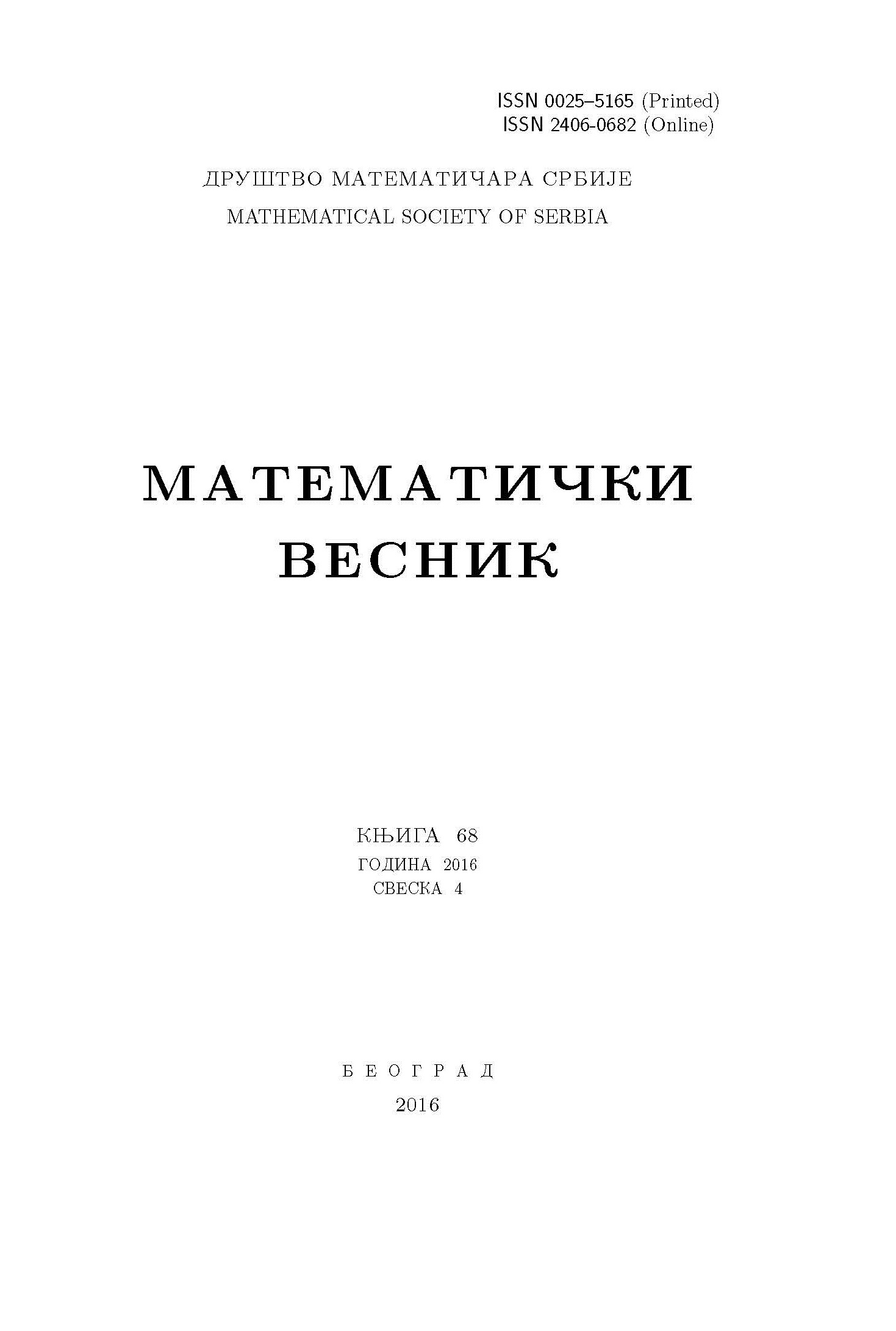﻿ Matematički Vesnik ﻿
 MATEMATIČKI VESNIK МАТЕМАТИЧКИ ВЕСНИКOPEN-POINT AND BI-POINT OPEN TOPOLOGIES ON CONTINUOUS FUNCTIONS BETWEEN TOPOLOGICAL (SPACES) GROUPS B. K. Tyagi, S. Luthra AbstractIn this paper, we study the notions of point-open topology $C_p(X,H)$, open-point topology $C_h(X,H)~[\text{resp.}~C_h(G,H)]$ and bi-point-open topology $C_{ph}(X,H)~[\text{resp.}$ $C_{ph}(G,H)]$ on $C(X,H)~[\text{resp.} C(G,H)]$, the set of all continuous functions from a topological space $X$ (topological group $G)$ to a topological group $H$. In this setting, we study the countability, separation axioms and metrizability. The equivalent conditions are given so that the space $C_h(G,H)$ is a zero-dimensional topological group. Further, if $G$ is $H^{\star\star}$-regular, then $C_h(G,H)$ is Hausdorff if and only if $G$ is discrete. It is shown that under certain conditions the topological groups $C_p(X,H)$, $C_h(X,H)$ and $C_{ph}(X,H)$ are $\omega$-narrow. Sufficient conditions are given for the topological spaces $C_p(X,H)$, $C_h(X,H)$ and $C_{ph}(X,H)$ to be discretely selective and to have a disjoint shrinking.Keywords: Point-open topology; open-point topology; bi-point-open topology; topological group; zero dimensional; $\omega$-narrow; disjoint shrinking; discrete selection. MSC: 54C35, 54A10, 54C05, 54D10, 54D15, 54E35, 54H11 Pages:  56$-$70 Volume  74 ,  Issue  1 ,  2022

﻿